# Eigenvalue and eigenvectors

### Eigenvalue and eigenvectors

#### Lessons

An eigenvector of an $n \times n$ matrix $A$ is a non-zero vector $x$ such that $Ax= \lambda x$, for some scalar $\lambda$. The scalar $\lambda$ is called the eigenvalue.

We say the eigenvector $x$ corresponds to the eigenvalue $\lambda$.

Given an eigenvalue $\lambda$ of matrix $A$, we can find a corresponding eigenvector $x$ by solving
$(A-\lambda I)x=0$
And finding a non-trivial solution $x$.

The eigenspace is the null space of the matrix $A-\lambda I$. In other words, the eigenspace is a set of all solutions for the equation
$(A-\lambda I)x=0$

Of course, we can find the basis for the eigenspace by finding the basis of the null space of $A-\lambda I$.
• Introduction
Eigenvalues and Eigenvectors Overview:
a)
Definition of Eigenvalues and Eigenvectors
• What are eigenvectors?
• What are eigenvalues?

b)
Verifying Eigenvalues/Eigenvectors of a Matrix
• Eigenvectors: Show that $Ax=\lambda x$
• Eigenvalues: Get a non-trivial solution for $(A-\lambda I)x=0$
• Finding a eigenvector given an eigenvalue

c)
Eigenspace
• What is an eigenspace?
• Finding a basis for the eigenspace

• 1.
Verifying Eigenvectors
Let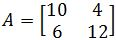. Is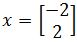an eigenvector of $A$? If so, find the eigenvalue. What about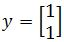?

• 2.
Let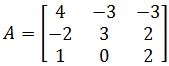. Is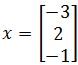an eigenvector of $A$? If so, find the eigenvalue.

• 3.
Verifying Eigenvalues and finding a corresponding eigenvector
Let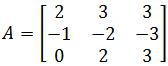. Is $\lambda=1$ an eigenvalue of $A$? If so, find a corresponding eigenvector.

• 4.
Finding a Basis for the Eigenspace
Find a basis for the corresponding eigenspace if: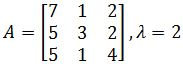• 5.
Proof Related to Eigenvalues and Eigenvectors
Prove that if $A^2$ is the zero matrix, then the only eigenvalue of $A$ is 0.

• 6.
Let $\lambda$ be an eigenvalue of an invertible matrix $A$. Then $\lambda ^{-1}$ is an eigenvalue of $A^{-1}$.